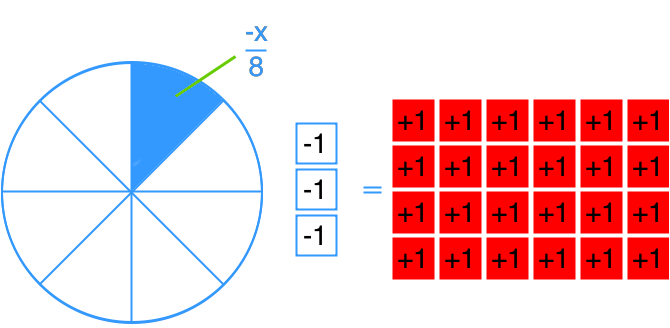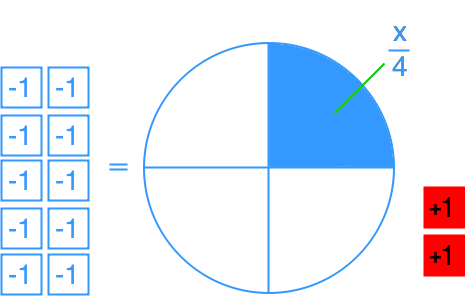# Solving two-step linear equations using multiplication and division: x/a + b = c#### All You Need in One Place

Everything you need for better marks in primary, GCSE, and A-level classes.#### Learn with Confidence

We’ve mastered the UK’s national curriculum so you can study with confidence.#### Instant and Unlimited Help

0/8
##### Examples
###### Lessons
1. Find x in the equation modelled by each diagram.

1.2.2. Solve.
1. $3 + \frac{x}{4} = 51$
2. $17 = 10 + \frac{x}{{ - 2}}$
3. $\frac{x}{{ - 6}} - 21 = - 9$
4. $- 1 = 23 + \frac{x}{8}$
3. The amount of time needed for an ice cube to melt when the temperature is between 10 °C to 30 °C can be expressed by the equation $t = 16 - \frac{C}{2}$, where t is the amount of time for the ice cube to melt in minute; and C is the temperature in degree Celsius.
1. Will the ice melt in 10 minutes if the temperature is 10 °C?
2. What was the lowest temperature for the ice cube to melt in 5 minutes?
0%
##### Practice
###### Topic Notes
Continue from the previous section, we will solve linear equations in diagram, equation, and word problems forms. However, in addition to subtraction and addition, we will teach you how to solve linear equations that also involve multiplications and divisions.